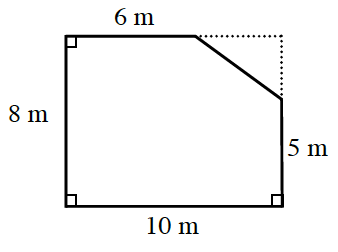### Home > ACC7 > Chapter 9 Unit 10 > Lesson CC3: 9.2.4 > Problem9-112

9-112.

Find the perimeter and area of the figure at right. Copy the figure on your paper, and show your work for each of the steps that you use.The shape to the right is missing a triangular segment in the top right corner.

With the inclusion of the triangular piece, the shape would form a rectangle.

Now solve for the area of the figure above by finding the area of the complete rectangle minus the area of the missing triangle piece.

With this information, we can solve for the lengths of the missing triangular piece:

$10 − 6 = 4$
$8 − 5 = 3$

$80 \text {m}^{2} − 6 \text {m}^{2} = 74 \text {m}^{2}$